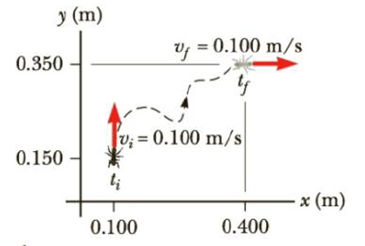Chapter 3, Problem 4P

Chapter
Section
Textbook Problem

An ant crawls on the floor along the curved path shown in Figure P3.4. The ant’s positions and velocities are indicated for times ti = 0 and tf = 5.00 s. Determine the x- and y-components of the ant’s (a) displacement, (b) average velocity, and (c) average acceleration between the two times.Figure P3.4

(a)

To determine
The x and y components of the ant’s displacement.

Explanation

Given info: The final x coordinate of the ant’s position is 0.400m , the initial x coordinate of the ant’s position is 0.100m , the final y coordinate of the ant’s position is 0.350m and the initial y coordinate of the ant’s position is 0.150m .

Explanation:

The x component of the ant’s displacement is,

Δx=xfxi

• xf is the final x coordinate
• xi is the initial x coordinate

Substitute 0.100m for xi and 0.400m for xf .

Δx=0.400m0.100m=0

(b)

To determine
The x and y components of the ant’s average velocity.

(c)

To determine
The x and y components of the ant’s average acceleration.

Still sussing out bartleby?

Check out a sample textbook solution.

See a sample solution

The Solution to Your Study Problems

Bartleby provides explanations to thousands of textbook problems written by our experts, many with advanced degrees!

Get Started